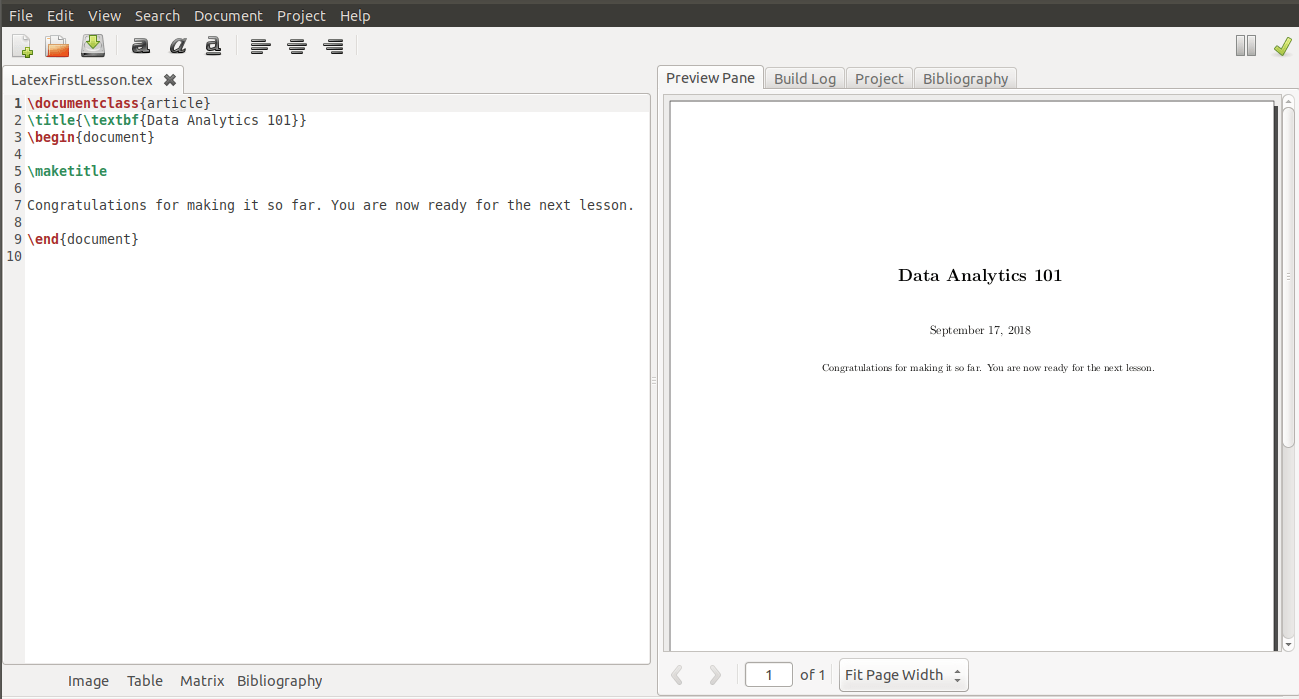# Referencing and Cross-referencing in Latex

### Article Summary

In this article, we can add a number of Latex snippets that are essential for citing external paper or resource (referencing) and citing internal items of the paper like a section, subsection, table or a figure.

### Latex Code Snippets

For adding references to your paper in Latex, there are two ways :

#### 1. Using \begin{bibliography}:

We add the References section always after the Conclusion section, at the end of the paper. We add each reference as a \bibitem, containing the name of a label(here, ref_lncs1). In order to mention this reference in the paper, we write: \cite{ref_lncs1}

\begin{bibliography}
\bibitem{ref_lncs1}
Author, F., Author, S.: Title of a proceedings paper. In: Editor, F., Editor, S. (eds.) CONFERENCE 2016, LNCS, vol. 9999, pp. 1--13. Springer, Heidelberg (2016). \doi{10.10007/1234567890}
\end{bibliography}

#### 2. Using BibTex :

\bibliography{file_name_bib}
\bibliographystyle{file_name_bst}

In case of a 2-column format, this will appear at the bottom of your column, separated by a line in case. In case of a single column format like Springer llcns, it will appear at the bottom of your page, separated by a single line.

To add a footnote inside the text, you use the command : “\footnote{text_content1}”

. This “text_content1” is usually an url, particularly a Github repo link.

#### 4. Adding references inside text :

To cite a paper, we write :

\ref{ref_lncs1}

as shown in  the code of point 1.

#### 5. Referencing tables and figures :

We use the \label tag to assign the name to the entity(maybe a table, figure or even a section), by which it will be called or referenced in different parts of the document. Let us for example consider the following templates, one for a table and second for a figure. Both of them make use of the \label tag.

##### 5.1 Table :
\begin{table}
\centering
\caption{Classifier performance results}
\label{table:classificationresults}
\begin{tabular}{|c|c|} \hline
Features (and Models)&amp;Accuracy (in \%)\\ \hline
Baseline 1 (Linear SVM using n-grams)&amp; 59.3\\
Baseline 2 &amp; 64.1\\
Our approach(lexicon based) &amp; 68.4\\
Ensemble SVM model (Optimal) &amp; 69.7\\
\hline\end{tabular}
\end{table}
##### 5.2 Figure :

I will later write an article on how to programmatically create Latex pictures using tikzpicture .

\begin{figure}
\centering
\includegraphics[width=\textwidth]{image_filename}
\caption{Workflow of our proposed methodology}
\label{fig:workflow}
\end{figure}
##### 5.3 Sections, subsections :
\section{Introduction}
\label{introduction}

#### 6. Examples in Latex used in our paper :

Here, instead of \cite which we previously showed for referencing bibliographic references, we will now use \ref to mention or reference a table, figure or section.

a) We show the performance of the classifier across different feature sets in Table~\ref{table:classificationresults}.
b) In Figure \ref{fig:workflow}, we provide a detailed workflow of our proposed methodology.
c) We motivate and outline the research challenges and the key contributions of this paper in Section \ref{introduction}.